Register a user on Elfpack

## Darkangel13000 (Saving Cereal.)

 Member #44038 created: 2006-08-15 21:39:10 Simple URL: http://www.elfpack.com/darkangel13000

Name: Courtney## [I love my Mary boo.... shes the cookies to my milk!]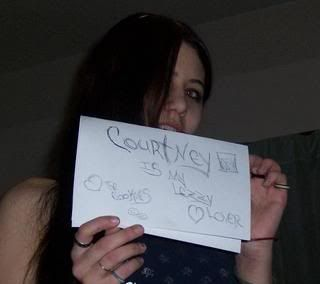Elfpack titles and orders

Description:
^^^^^^^^^^^^^^^^^ 8^^^^^^^^^^^^^^^P
^^^^^^^^^^8 ^^8^^88^^^^^^^^^^^^^^^S
^^^^^ ^^^^^88^8^888^888^^^^^^^^^^^^Y
^^^^^^^^^^^8888888888^^^^^^^ ^^^^^^^C
^^^^^^^^888888888888 8^^^^^8888888^^^^H
^^^^^^^^^^ 888888888888^^^^^8888888^^O
^ ^^^^^^^8888888888888^^^^^^^888 8888^^p
^^^^^^^^^^^8888888888 ^^^^^^8888888^^A
^^^^^^^^^^^8 ^^88^^888^^^^88888888^^T
^^^^ ^^^^^^^^^^88^^^^^^^^^^^88888^^ H
^^^^^^^^^888888888888^^^^^^ 888^88^^^^I
^^^^^^^8888^^8888 8888888^8888^^^88^^^^C
^^^^^^ 888^^^^8888888^^88888^^^^88^^^ ^^
^^^^^888^^^^^8888888^^^^^^ ^^^^^^^^^^^H
^^^^8888^^^^^888 88888^^^^^^^^^^^^^^^^^A
^^^^^ 8 88^^^^^8888888^^^^^^^^^8888^^^ ^T
^^^^^^^^^^^^^88888888^^^88 8888888^^^^C
^^^^^^^^^^^^^888 88888888888^^^^888^^^H
^^^^^^ ^^^^^^^88888888^^^^^^^^^888^^^ ^E
^^^^^^^^^^^^^888^^^^^^^^^^ ^^8888^^^^^T
^^^^^^^^^^^^888^ ^^^^^^^^^^^888888^^^^M
^^^^^8 88^^^888^^^^^^^^^^^^^^8888888^ ^A
^^^^88888^888^^^^^^^^^^^^^ ^^^^^^^^^^^N
^^8888^^8888^^^^ ^^^^^^^^^^^^^^^^^^^^^
*Juggalo Pledge*
I pledge allegiance to the Hatchet of the Underground Juggalo Society,
and to the Ninjas for which it stands,
One Family,
Under Clowns,
Full of Freaks,
with Faygo and Magik Neden for all!# So I'm hardly on here so if you really want to talk Message me on myspace yo! www.myspace.com/420_courtney

## I <3 My Friends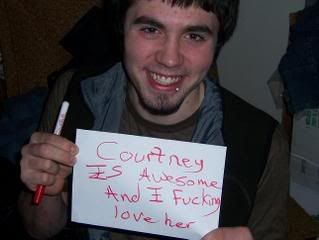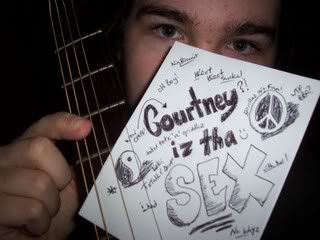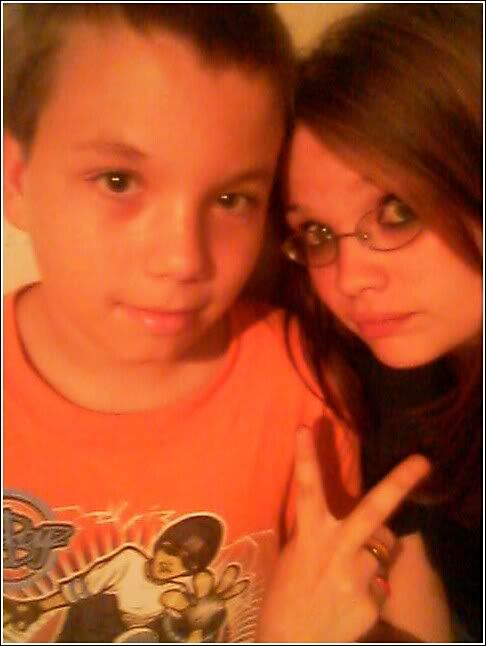# Keppin it[gangster]# Killswitch Engage concert 12/8/06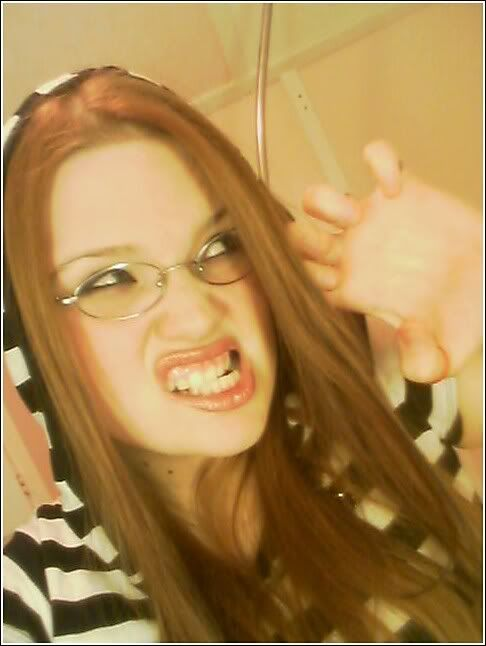[

## FEAR ME!

]

 Age: 27 Year of birth: 1991 Month of birth: 4 Day of birth: 20

Gender: female

What do you do?: Something in between

Place of living: USA-Michigan

Exact place of living: Battle Creek

Known languages
English

Music
alternativebluescountry
gothgrungeheavy metal
hip hoppoppunk
raprocktechno

Other interests
animalsartcard games
catsdancingdogs
drinkseatinghorses
partypoetrysinging
smokingshopping

Civil status: single

Sexual preference: opposite sex

Body shape: normal

Height: 163